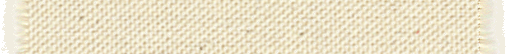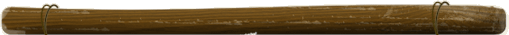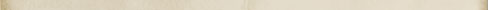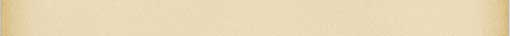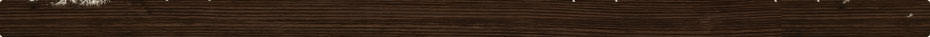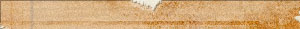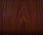# as2js: as2js::optimizer_details Namespace Reference

as2js  0.1.14
AlexScript to JavaScript
as2js::optimizer_details Namespace Reference

The optimizer sub-namespace. More...

Namespaces  anonymous_namespace{optimizer_matches.cpp}  Hide all optimizer compare implementation details.
anonymous_namespace{optimizer_optimize.cpp}  Hide all optimizer "optimize function" implementation details.
anonymous_namespace{optimizer_tables.cpp}  Hide all optimizer tables implementation details.
Classes struct  optimization_entry_t   struct  optimization_match_t   struct  optimization_optimize_t   struct  optimization_table_t   struct  optimization_tables_t   Typedefs typedef uint16_t index_t   Enumerations enum  optimization_function_t {
optimization_function_t::OPTIMIZATION_FUNCTION_BITWISE_AND,
optimization_function_t::OPTIMIZATION_FUNCTION_BITWISE_NOT,
optimization_function_t::OPTIMIZATION_FUNCTION_BITWISE_OR,
optimization_function_t::OPTIMIZATION_FUNCTION_BITWISE_XOR,
optimization_function_t::OPTIMIZATION_FUNCTION_COMPARE,
optimization_function_t::OPTIMIZATION_FUNCTION_CONCATENATE,
optimization_function_t::OPTIMIZATION_FUNCTION_DIVIDE,
optimization_function_t::OPTIMIZATION_FUNCTION_EQUAL,
optimization_function_t::OPTIMIZATION_FUNCTION_LESS,
optimization_function_t::OPTIMIZATION_FUNCTION_LESS_EQUAL,
optimization_function_t::OPTIMIZATION_FUNCTION_LOGICAL_NOT,
optimization_function_t::OPTIMIZATION_FUNCTION_LOGICAL_XOR,
optimization_function_t::OPTIMIZATION_FUNCTION_MATCH,
optimization_function_t::OPTIMIZATION_FUNCTION_MAXIMUM,
optimization_function_t::OPTIMIZATION_FUNCTION_MINIMUM,
optimization_function_t::OPTIMIZATION_FUNCTION_MODULO,
optimization_function_t::OPTIMIZATION_FUNCTION_MOVE,
optimization_function_t::OPTIMIZATION_FUNCTION_MULTIPLY,
optimization_function_t::OPTIMIZATION_FUNCTION_NEGATE,
optimization_function_t::OPTIMIZATION_FUNCTION_POWER,
optimization_function_t::OPTIMIZATION_FUNCTION_REMOVE,
optimization_function_t::OPTIMIZATION_FUNCTION_ROTATE_LEFT,
optimization_function_t::OPTIMIZATION_FUNCTION_ROTATE_RIGHT,
optimization_function_t::OPTIMIZATION_FUNCTION_SET_INTEGER,
optimization_function_t::OPTIMIZATION_FUNCTION_SET_NODE_TYPE,
optimization_function_t::OPTIMIZATION_FUNCTION_SHIFT_LEFT,
optimization_function_t::OPTIMIZATION_FUNCTION_SHIFT_RIGHT,
optimization_function_t::OPTIMIZATION_FUNCTION_SHIFT_RIGHT_UNSIGNED,
optimization_function_t::OPTIMIZATION_FUNCTION_SMART_MATCH,
optimization_function_t::OPTIMIZATION_FUNCTION_STRICTLY_EQUAL,
optimization_function_t::OPTIMIZATION_FUNCTION_SUBTRACT,
optimization_function_t::OPTIMIZATION_FUNCTION_SWAP,
optimization_function_t::OPTIMIZATION_FUNCTION_TO_CONDITIONAL,
optimization_function_t::OPTIMIZATION_FUNCTION_TO_INT64,
optimization_function_t::OPTIMIZATION_FUNCTION_TO_NUMBER,
optimization_function_t::OPTIMIZATION_FUNCTION_WHILE_TRUE_TO_FOREVER
}   Functions void apply_functions (node_pointer_vector_t &node_array, optimization_optimize_t const *optimize, size_t optimize_size)  Apply all the optimization functions. More...
bool match_tree (node_pointer_vector_t &node_array, Node::pointer_t node, optimization_match_t const *match, size_t match_count, uint8_t depth)  Compare a node against an optimization tree. More...
bool optimize_tree (Node::pointer_t node)  Optimize a tree of nodes as much as possible. More...
Variables uint32_t const OPTIMIZATION_ENTRY_FLAG_UNSAFE_MATH = 0x0001   uint32_t const OPTIMIZATION_ENTRY_FLAG_UNSAFE_OBJECT = 0x0002   uint32_t const OPTIMIZATION_MATCH_FLAG_CHILDREN = 0x0001   Detailed Description

This namespace is used to define all the optimizer internal tables, functions, and classes.

We have a separate namespace because the number of tables in the optimizer is really large and could clash with other parts of the libraries.

Typedef Documentation

Definition at line 147 of file optimizer_tables.h.

Enumeration Type Documentation
EnumeratorOPTIMIZATION_FUNCTION_ADD  OPTIMIZATION_FUNCTION_BITWISE_AND  OPTIMIZATION_FUNCTION_BITWISE_NOT  OPTIMIZATION_FUNCTION_BITWISE_OR  OPTIMIZATION_FUNCTION_BITWISE_XOR  OPTIMIZATION_FUNCTION_COMPARE  OPTIMIZATION_FUNCTION_CONCATENATE  OPTIMIZATION_FUNCTION_DIVIDE  OPTIMIZATION_FUNCTION_EQUAL  OPTIMIZATION_FUNCTION_LESS  OPTIMIZATION_FUNCTION_LESS_EQUAL  OPTIMIZATION_FUNCTION_LOGICAL_NOT  OPTIMIZATION_FUNCTION_LOGICAL_XOR  OPTIMIZATION_FUNCTION_MATCH  OPTIMIZATION_FUNCTION_MAXIMUM  OPTIMIZATION_FUNCTION_MINIMUM  OPTIMIZATION_FUNCTION_MODULO  OPTIMIZATION_FUNCTION_MOVE  OPTIMIZATION_FUNCTION_MULTIPLY  OPTIMIZATION_FUNCTION_NEGATE  OPTIMIZATION_FUNCTION_POWER  OPTIMIZATION_FUNCTION_REMOVE  OPTIMIZATION_FUNCTION_ROTATE_LEFT  OPTIMIZATION_FUNCTION_ROTATE_RIGHT  OPTIMIZATION_FUNCTION_SET_INTEGER  OPTIMIZATION_FUNCTION_SET_NODE_TYPE  OPTIMIZATION_FUNCTION_SHIFT_LEFT  OPTIMIZATION_FUNCTION_SHIFT_RIGHT  OPTIMIZATION_FUNCTION_SHIFT_RIGHT_UNSIGNED  OPTIMIZATION_FUNCTION_SMART_MATCH  OPTIMIZATION_FUNCTION_STRICTLY_EQUAL  OPTIMIZATION_FUNCTION_SUBTRACT  OPTIMIZATION_FUNCTION_SWAP  OPTIMIZATION_FUNCTION_TO_CONDITIONAL  OPTIMIZATION_FUNCTION_TO_INT64  OPTIMIZATION_FUNCTION_TO_NUMBER  OPTIMIZATION_FUNCTION_WHILE_TRUE_TO_FOREVER

Definition at line 102 of file optimizer_tables.h.

Function Documentation
void as2js::optimizer_details::apply_functions ( node_pointer_vector_tnode_array, optimization_optimize_t const *  optimize, size_t  optimize_size  )

This function applies all the optimization functions on the specified array of nodes one after the other.

If a parameter (node) is invalid for a function, an exception is raised. Because the optimizer is expected to properly match nodes before an optimization can be applied, the possibility for an error here should be zero.

Parameters
[in]node_arrayThe array of nodes as generated by the matching code. [in]optimizePointer to the first optimization function. [in]optimize_sizeThe number of optimization functions to apply.

Definition at line 2070 of file optimizer_optimize.cpp.

bool as2js::optimizer_details::match_tree ( node_pointer_vector_tnode_array, Node::pointer_t  node, optimization_match_t const *  match, size_t  match_count, uint8_t  depth  )

This function goes through a node tree and and optimization tree. If they both match, then the function returns true.

The function is generally called using the node to be checked and the match / match_count parameters as found in an optimization structure.

The depth is expected to start at zero.

The function is recursive in order to handle the whole tree (i.e. when the function determines that the node is a match with the current match level, it then checks all the children of the current node if required.)

Parameters
[in]node_arrayThe final array of nodes matched [in]nodeThe node to be checked against this match. [in]matchAn array of match definitions. [in]match_countThe size of the match array. [in]depthThe current depth (match->f_depth == depth).
Returns
true if the node matches that optimization match tree.

Definition at line 327 of file optimizer_matches.cpp.

bool as2js::optimizer_details::optimize_tree ( Node::pointer_t  node)

This function checks the specified node against all the available optimizations defined in the optimizer.

Todo:
Look into losing the recusirve aspect of this function (so the entire tree of nodes gets checked.)
Parameters
[in]nodeThe node being checked.
Returns
true if any optimization was applied

Definition at line 213 of file optimizer_tables.cpp.

Referenced by as2js::Optimizer::optimize().

Variable Documentation
uint32_t const as2js::optimizer_details::OPTIMIZATION_ENTRY_FLAG_UNSAFE_MATH = 0x0001

Definition at line 156 of file optimizer_tables.h.

uint32_t const as2js::optimizer_details::OPTIMIZATION_ENTRY_FLAG_UNSAFE_OBJECT = 0x0002

Definition at line 157 of file optimizer_tables.h.

uint32_t const as2js::optimizer_details::OPTIMIZATION_MATCH_FLAG_CHILDREN = 0x0001

Definition at line 73 of file optimizer_tables.h.

Referenced by match_tree().

This document is part of the Snap! Websites Project.